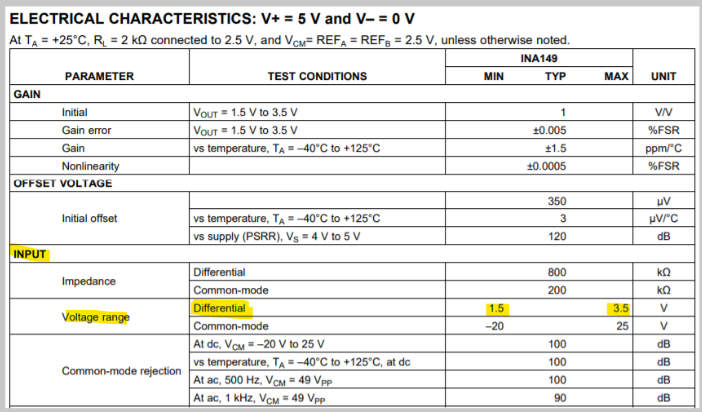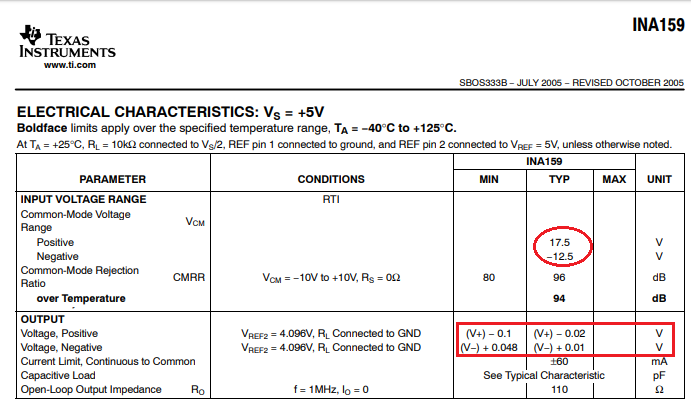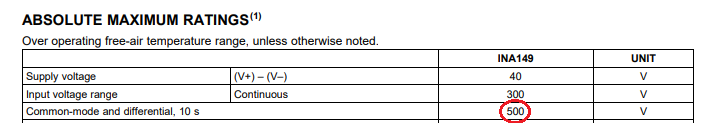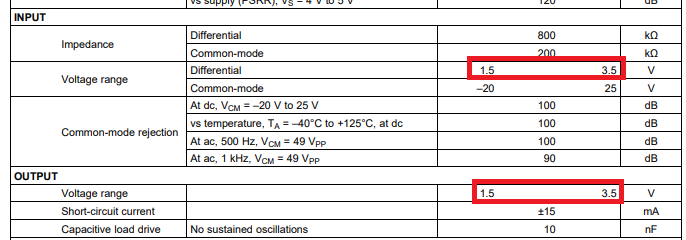If you have a related question, please click the "Ask a related question" button in the top right corner. The newly created question will be automatically linked to this question.

• TI Thinks Resolved

# INA149: INA149 Operating Limits

Part Number: INA149

I am using INA149 and have some questions about its operating limits.

I am using it with the following inputs : V+ = 5 V and V– = 0 V, REFA = REFB = 2.5 V

My question is what is the differential voltage rated to? I plan on having a voltage difference of up to 15V for short period of time (<1sec). Can it survive this with no damage? What would Vout’s value be?

• Hi Marshall,

The INA149 is a high common mode voltage difference amplifier. On a 5V single supply with REFA=REFB=2.5V, the input differential voltage allowed is 1.5V to 3.5V for linear operation. That is 1.5V from each rail.

The common mode voltage is the voltage that is the same on both inputs, and the differential voltage is the difference between the two inputs. The INA149 can handle high common mode voltages (+/-275V).

Assume you have a single supply 5V with REFA = REFB = 2.5V. You can have one input at 100V and the other at 103V with no issues. That's because the differential voltage is 3V (and that's within the allowable range I shared earlier), and the common mode voltage is 100V which is <275V.

If you need to sense a voltage difference up to 15V, you will need to increase your supplies.The absolute maximum ratings for this device can be found on page 2 of the datasheet. The absolute maximum common mode and differential voltage is 500V. But it is important to note that we do not recommend operating at these conditions for extended periods as this may degrade device performance and reliability.

-Tamara

-Tamara Alani

• However, INA149 is a high common-mode difference amplifier with a gain of 1. This means that it is meant to be used for high-input common-mode voltages with the linear input differential signal being limited by the output voltage swing - see below.  For that reason, a better solution for 5V single supply may be to use INA159 with gain of 0.2, which will allow higher differential input voltage - see below.Marek Lis, MGTS
Sr Application Engineer
Precision Analog - TI Tucson

• In reply to Tamara M Alani:

Hello,

According to the max rating the differential voltage is 500V. So, I am wondering if the differential does go above 3.5V. will the part be protected up to 500V? Will it just output the max it can? 3.5V for my case I believe.

• In reply to Marshall Dorin:

Marshall,

A short answer is yes.  As long as input common-mode or differential voltage is less than 500V, the part will not be damage.Depending on the polarity of the input differential voltage, the output will be pushed against either positive or negative rail (<1.5V or >3.5V).Marek Lis, MGTS
Sr Application Engineer
Precision Analog - TI Tucson

• In reply to Marek Lis:

So if the input differential is less than 1.5V it will be clipped at 1.5V and if it is greater than 3.5V it will be clipped at 3.5V? but as long as it is below 500V differential there will be no damage?

• In reply to Marshall Dorin:

Where the output will be clipped depends on the reference voltage.

For Vref=2.5V and Vin_diff >1V Vout will be clipped above 3.5V

For Vref=2.5V and Vin_diff <-1V Vout will be clipped below 1.5V

For Vref=0 and Vin_diff < 1.5V Vout will be clipped below 1.5V

For Vref=5V and Vin_diff >-1.5V Vout will be clipped above 3.5V

For Vref=0 and Vin_diff > 3.5V Vout will be clipped above 3.5V

For Vref=5V and Vin_diff <-3.5V Vout will be clipped below 1.5V

As long as the Vin_diff is less than 500V for 10s or less, INA149 will not be damaged.

Marek Lis, MGTS
Sr Application Engineer
Precision Analog - TI Tucson

• In reply to Marek Lis:

Hi Marek,

Sorry I'm a little confused by this. According the page 4 of the data sheet. the input of the differential voltage range is 1.5V to 3.5V with 2.5V ref.

However it looks like based on what you just sent is that those values are for Vref = 0. and when Vref is 2.5V it is actually between -1V to 1V.

thank you,

• In reply to Marshall Dorin:

That's exactly correct.  The output must be between 1.5V to 3.5V on 5V single supply.  Therefore, since the Gain is 1, for Vref of 2.5V, the maximum Vin_diff voltage you may apply is +/-1V, so the output Vout=Vref+/-Vin_diff*Gain = 2.5V+/-1V*1 = 1.5 to 3.5V

Marek Lis, MGTS
Sr Application Engineer
Precision Analog - TI Tucson